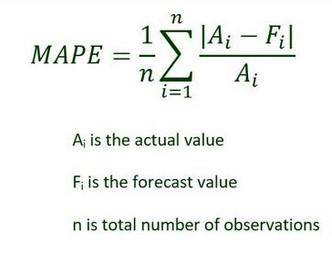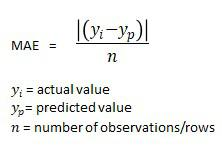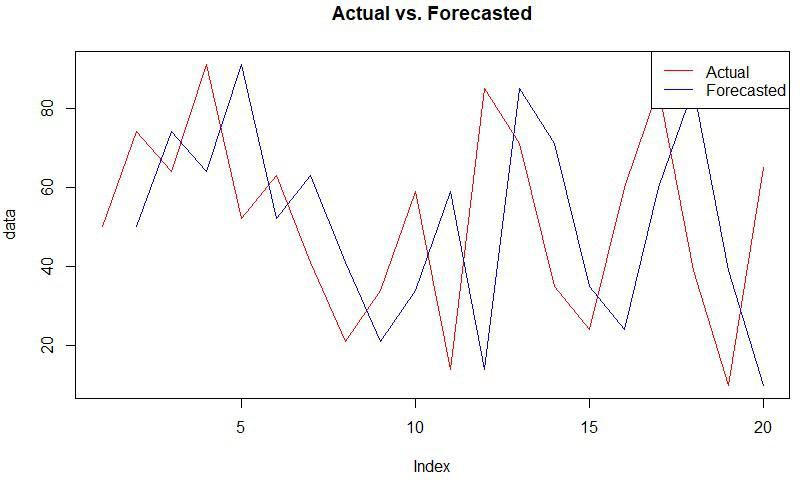Open in App
Not now

# How to Perform Naive Forecasting in R

• Last Updated : 14 Dec, 2022

In this article, we are going to learn how to perform naive forecasting in the R programming language.

### Naive Forecasting

A naïve forecast is one where the value predicted for a certain period is the same as seen in the preceding period. This is a method of estimation that uses the last period’s actuals as the forecast for a current period without making any adjustments or attempting to identify the causes. It is solely used to compare forecasts made using more advanced (better) approaches.

## The step-wise procedure to perform naive forecasting:

Step 1: In this step, we are simply providing the data in the form of a vector containing 20 integers and storing it in a variable; this is the actual data, which will further be used to perform naive forecasting.

## R

 `# Creating data``data <-``c``(50,74,64,91,52,63,41,``21,34,59,14,85,71,35,24,60,85,39,10,65)``# Printing Data``print``(data)`

Output:

`  50 74 64 91 52 63 41 21 34 59 14 85 71 35 24 60 85 39 10 65`

Step 2: In this step, we simply put the actual data into the formula to create naive forecasts for each corresponding data.

The syntax for the forecast formula:

x <- c(NA,data[-length(data)])

here, data is the actual data which is used to forecast and  NA is used for the first forecasted value.

## R

 `data <-``c``(50,74,64,91,52,63,41,21,``34,59,14,85,71,35,24,60,85,39,10,65)``# Using the naive formula to get the``# forecast and storing the values in variable``forecast <- ``c``(``NA``, data[-``length``(data)])``# Printing forecast``print``(forecast)`

Output:

`  NA 50 74 64 91 52 63 41 21 34 59 14 85 71 35 24 60 85 39 10`

Step 3:In this step, we are measuring the accuracy of the generated forecasts in step 2; here, we will calculate the accuracy using mean absolute percentage error(MAPE) and mean absolute error(MAE).

Mean absolute percentage error(MAPE):

The average or mean of forecasts’ absolute percentage error is the mean absolute percentage error (MAPE). Actual or observed value less predicted value is the definition of error. To calculate MAPE, percentage errors are added without regard to sign.Mean absolute error(MAE):

This determines the average error size in a batch of predictions without taking direction into account. It represents the weighted average of the individual deviations between the actual observation and the forecast over the test sample.## R

 `data <-``c``(50,74,64,91,52,63,41,21,``34,59,14,85,71,35,24,60,85,39,10,65)``forecast <- ``c``(``NA``, data[-``length``(data)])` `# Calculating mean absolute percentage error``print``(``"MAPE"``)``mean``(``abs``((data-forecast)/data), na.rm=T) * 100` `# Calculating mean absolute error``print``(``"MAE"``)``mean``(``abs``(data-forecast), na.rm=T)`

Output:

``` "MAPE"
 81.84397
 "MAE"
 29.42105```

Step 4:In this step, we will visualize the Forecasts using the plot() and lines() function; here, we will create a simple line plot to visualize the differences between the actual sales and the naive forecasts during each period.

## R

 `data <-``c``(50,74,64,91,52,63,41,21,34,``   ``59,14,85,71,35,24,60,85,39,10,65)``forecast <- ``c``(``NA``, data[-``length``(data)])` `# Plotting the actual points``# and the forecast points``plot``(data, type=``'l'``, col = ``'red'``,``     ``main=``'Actual vs. Forecasted'``)``lines``(forecast, type=``'l'``, col = ``'blue'``)``legend``(``'topright'``, legend=``c``(``'Actual'``,`` ``'Forecasted'``),col=``c``(``'red'``, ``'blue'``), lty=1)`

Output:My Personal Notes arrow_drop_up